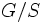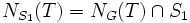# Finding conjugate subgroups

This is a survey article related to:conjugacy
View other survey articles about conjugacy

• Prove that two subgroups of a group are conjugate. In other words, given a group$G$ and two subgroups$H$ and$K$ of$G$, we need to show the existence of a$g \in G$ suchthat$gHg^{-1} = K$
• Prove a domination condition: Show that a given subgroup is conjugate to some subgroup of another given subgroup. In other words, given a group$G$ and subgroups$H$ and$K$, we want to show that there exists$g \in G$ such that$gHg^{-1} \le K$
• Prove the existence of another conjugate subgroup, with somewhat nicer properties

## Move the big, not the small

It is helpful to keep in mind that the following are equivalent for subgroups$H,K$ of$G$:

1. A conjugate of$H$ is contained in$K$
2.$H$ is contained in a conjugate of$K$

It is usually the second form that is more useful. In other words, it is usually more helpful to try to find a conjugate of$K$ containing$H$, rather than try to find a conjugate of$H$ contained in$K$. In some sense, the bigger groups are easier to move around than the smaller groups.

A formulation equivalent to (2) is:

• The action of$H$ on the coset space$G/K$ has a fixed point

### Proving the domination in Sylow's theorem

Further information: Sylow's theorem#Proof of domination

One part of Sylow's theorem states if for for a finite group$G$, a$p$-Sylow subgroup$S$ of$G$ and a$p$-subgroup$H$ of$G$,$H$ is conjugate to a subgroup of$S$.

We now consider the action of$H$ on the quotient space$G/S$, by left multiplication. Since the order of$G/S$ is relatively prime to$p$, there is some orbit whose order is not a multiple of$p$, but since$H$ is a$p$-group, this orbit must have size one. Thus, the action of$H$ on the quotient space$G/S$ has a fixed point, so$H$ is contained in the conjugate of$S$ that fixes that point.

### Proving that any finite subgroup is contained in the orthogonal group

Further information: Orthogonal group is finite-dominating in general linear group

Another example is the following: given a finite subgroup of$GL(n,\R)$ (the general linear group), there exists a conjugate of it contained in$O(n,\R)$ (the orthogonal group). In other words, the orthogonal group dominates all finite groups.

Again, it is easier instead to prove the following: given any finite subgroup of$GL(n,\R)$, there exists a conjugate of the orthogonal group containing it. Saying that a group is contained in a conjugate of the orthogonal group, is equivalent to saying that there exists a positive definite symmetric bilinear form invariant under the action of the group. Thus, the problem reduces to the following: given any finite subgroup of$GL(n,\R)$, find a positive-definite symmetric bilinear form invariant under the group.

This is achieved by taking any positive-definite symmetric bilinear form, and then averaging it over the group.

### Proving that any finite subgroup of diffeomorphism group has an invariant Riemannian metric

We can take this somewhat further: given any finite subgroup of the self-diffeomorphism group of a differential manifold, there exists a Riemannian metric on the differential manifold that is invariant under the finite group. In other words, there is a Riemannian metric such that the elements of the finite group are isometries for that Riemannian metric.

The proof is exactly the same as the previous one: we start with any Riemannian metric, and average under the action of the finite group. The key difference from the preceding case is that in the previous case, all the isometry groups for positive-define symmetric bilinear forms were conjugate. Now, it is no longer true that isometry groups of Riemannian metrics are all conjugate, so we cannot obtain a single group that is finite-dominating.

## Move the big, and Sylow's theorem

We often need to combine the basic results of Sylow theory, with the idea that it's the bigger subgroup that needs to be moved around. Below are some examples.

### Moving to nice p-subgroups

The setup is like this:$G$ is a finite group,$S$ is a$p$-Sylow subgroup, and$T$ is a subgroup of$G$ contained inside$S$. Then, we need to find a subgroup$U$ of$G$ such that$T$ and$U$ are conjugate inside$G$, and such that$N_S(U)$ is a$p$-Sylow subgroup inside$N_G(U)$.

The key trick here is again, to focus attention on trying to move the big rather than the small. So, instead of chasing$U$, we look at the subgroup$N_G(T)$. Let$V$ be a$p$-Sylow subgroup of$N_G(T)$. Now, by the domination part of Sylow's theorem,$V$ is contained in some conjugate$S_1$ of$S$ (i.e. in another Sylow subgroup). Let us try to determine what$N_{S_1}(T)$ looks like.

Clearly,$N_{S_1}(T) = N_G(T) \cap S_1$. This contains$V$, because$V$ is contained in both subgroups. Also, it cannot be strictly bigger than$V$, because$V$ is Sylow inside$N_G(T)$.

So what we've done is: instead of moving$T$ to a good subgroup of$S$, we've found a Sylow subgroup$S_1$ in which$T$ is good. Now, of course, we can do a conjugation taking$S_1$ to$S$ and the image of$T$ under that conjugation is the required$U$.